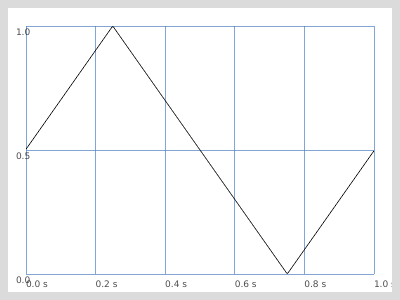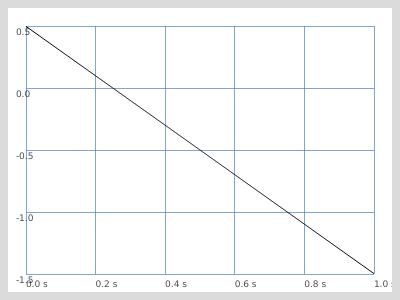# Negative frequency values for LFTri

Dear list,

I am trying to use an LFTri for outputting values between 0.0 and 1. The frequency of the oscillator will vary between positive and negative values in the range between -3.0 to 3.0. However, when LFTri takes a negative frequency value, it eventually outputs increasingly negative values, regardless of the scaling.
SinOsc will stay in the range of 0-1, even with negative frequency values.
I have two solutions, none of which are ideal:

1. Use SinOsc. This is problematic, as I want a linear ramp.
2. Convert the frequency values into absolute values. I would like to make the oscillator be able to ramp in both directions, between 0 -1 and 1-0, which makes this solution problematic.

Any ideas? Is this because of the non-bandlimited nature of LFTri?

Here’s a test:

``````{LFTri.kr(-0.1,0, 0.5, 0.5).poll}.play // Goes way below 0
{SinOsc.kr(-0.1,0, 0.5, 0.5).poll}.play // stays in the range of 0-1

``````

It looks like most LFUGens (LFTri, LFPar, LFGauss, LFPulse, LFCub … all of them except LFSaw if I’m not wrong) are not reading backwards when given a negative frequency.
Here are some plots of what I get with LFTri:
`{LFTri.kr(1).range(0,1)}.plot(1)``{LFTri.kr(-1).range(0,1)}.plot(1)`Looking in the source code, while LFSaw explicity checks for negative frequencies, the others aren’t. I think this could be a feature request.

In the meanwhile, depending on what you need to do, you could maybe get away with some other ways to get what you need. For example, a phase value of 2 produces the exact “reverse” of an LFTri.
`LFTri.kr(1,2).range(0,1)`

Or you could write the wave to a Buffer and read it with BufRd

Cheers

Hi Gianluca!

Thanks for the reply. Yes, that makes sense. Both of your suggestion are good, I’ll try them.

Then maybe you’ll like these two ways to get a triangle wave that you can modulate to negative freqs:

``````// 1. Wavetable (for Osc UGen)
// define the triangle wave as an Env. convert to wavetable, load to buffer
Env([0,1,0,-1,0],0.25).asSignal(1024).asWavetable
);
// play buffer with osc: it has a nice frequency control
{Osc.kr(~tri,-1)}.plot(1)

// 2. get a triangle wave from a Phasor, also nice freq ctrl
{
var freq = -1;
var numSamples = ControlRate.ir;
Phasor.kr(1,freq,0,numSamples).fold2(numSamples/4) / (numSamples/4)
}.plot(1)
``````
1 Like

I used the phasor solution and it works great, thanks!

1 Like